Oct 17, 2014
8014 Views
2 0

# Grade 2: Maths Worksheets Part 1This section includes maths worksheets for Grade 2. For maths it is a must to practice as much as your child can. Let them avail these worksheets and improve their concepts.

TopicDescriptionSample WorksheetSet of worksheets (All free)
Thousands< Hundreds, Tens and Units - 4 Digits (Blocks)Thousands, Hundreds, Tens and Units. Placing value of numbers to 9999.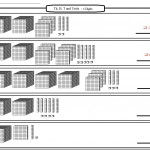Thousands, Hundreds, Tens and Units Worksheets
Reading and Writing Numbers - 4 DigitsSet 1 - Writing from words to numbers and from numbers to words.
Set 2 - Circle the correct digit and write the value of given digit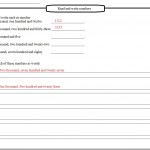Set 1 Reading and Writing Numbers Worksheets
Set 2 Reading and Writing Numbers Worksheets
Using an Abacus for 5 Digit NumbersWriting numbers shown on abacus and how to place beads on an abacus to represent numbers.Using an Abacus for 5 Digit Numbers Worksheets
Subtracting 3 Digit Numbers: No renamingSubtracting 456 - 112 = 344 but not using Units, Tens and HundredsSubtracting 3 Digit Numbers: No renaming Worksheets
Rounding to the nearest 10 and 100Rounding off numbers to nearest 10 for example 183 would be 180 and rounding off to the nearest 100 where 183 would be 200.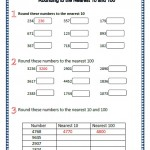Rounding Off to the nearest 10 and 100 Worksheets
Reading and Writing Numbers 5 DigitsReading and writing numbers in words.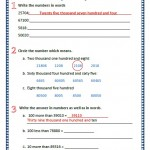Reading and Writing 5 Digit Numbers Worksheets
Putting Numbers in OrderPutting the numbers smallest to largest
For example 197, 134, 143 would be 134, 143 and 197. Then largest to smallest for the same question would be 197, 143 and 134.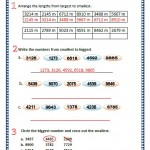Putting Numbers in OrderWorksheets
Place Value: 5 Digit NumbersPlacing values of 5 digit numbers. Like in 23467 the number 3's value would be 3 thousand.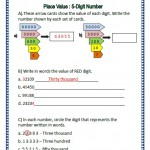Place Value 5 Digit Numbers Worksheets
Adding Numbers RenamingRenaming of number means using Units, Tens Hundreds, Thousands and so on for addition purpose.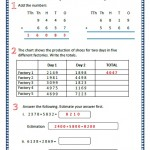Adding Numbers Renaming Worksheets
Adding 4 Digit Numbers: No RenamingNo renaming means not using Units, Tens, Hundreds and so on for addition.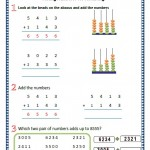Adding Numbers: No renaming Worksheets
Adding 3 NumbersAdding 3 numbers would be 2341 + 2222 + 1221 = 5784.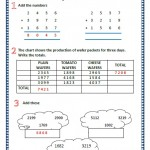Adding 3 Numbers Worksheets
Adding 3 Digit NumbersAddition of 3 Digits like 351 + 548 = 899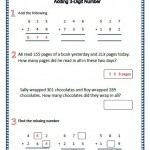Adding 3 Digit Numbers Worksheets
Comparing Numbers Comparing Numbers using sign "<" or ">"Comparing Numbers Worksheets
Subtracting 4 Digit Numbers: No Renaming
Subtracting 3 Digit Numbers: With Exchange
Subtracting 4 Digit Numbers: Renaming
Number Properties and Pattern
Counting 10's, 100's and 1000's
Even and Odd
Number Pattern
Patterns and ProblemsCounting in groups and writing
TimePutting months in order and focusing on hours, days, weeks and months
Minutes Past HourWriting the time on the clocks as minutes past the hour. Drawing the given time on the clock face and showing the time on the clock faces.

More topics would be adding up soon.

Article Categories:
Grade 2 Worksheets · Maths Worksheets